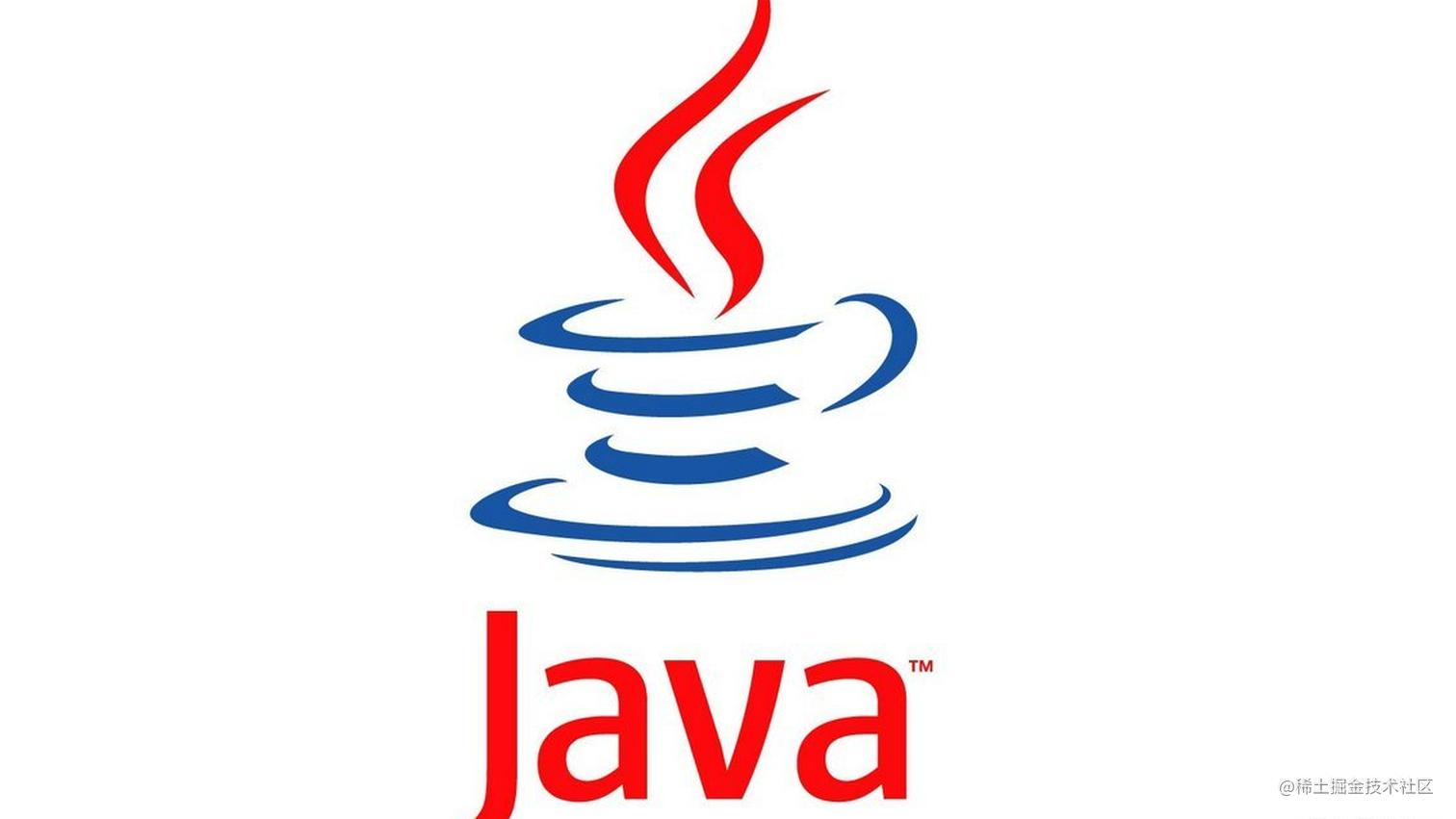# 别傻傻不知道 == 和 equals 的区别【面试系列】## == 是什么？

`==`常用于相同的基本数据类型之间的比较，也可用于相同类型的对象之间的比较；

• 如果 `==`比较的是基本数据类型，那么比较的是两个基本数据类型的值是否相等；

• 如果 `==`是比较的两个对象，那么比较的是两个对象的引用，也就是两个对象是否为同一个对象，并不是比较的对象的内容；

``public class Test {    public static void main(String[] args){        // 对象比较        User userOne = new User();        User userTwo = new User();        System.out.println("userOne==userTwo : "+(userOne==userTwo));        // 基本数据类型比较        int a=1;        int b=1;        char c='a';        char d='a';        System.out.println("a==b  :  "+(a==b));        System.out.println("c==d  :  "+(c==d));    }}复制代码``

``class User {     String userName;     String password;}复制代码``

``userOne==userTwo : falsea==b  :  truec==d  :  true复制代码``

## equals 是什么鬼？

equals方法主要用于两个对象之间，检测一个对象是否等于另一个对象；

``public boolean equals(Object obj) {        return (this == obj);    }复制代码``

java语言规范要求equals方法具有以下特性：

• 自反性。对于任意不为null的引用值x，x.equals(x)一定是true。

• 对称性）。对于任意不为null的引用值x和y，当且仅当x.equals(y)是true时，y.equals(x)也是true。

• 传递性。对于任意不为null的引用值x、y和z，如果x.equals(y)是true，同时y.equals(z)是true，那么x.equals(z)一定是true。

• 一致性。对于任意不为null的引用值x和y，如果用于equals比较的对象信息没有被修改的话，多次调用时x.equals(y)要么一致地返回true要么一致地返回false。

• 对于任意不为null的引用值x，x.equals(null)返回false。

• String

``public boolean equals(Object anObject) {        if (this == anObject) {            return true;        }        if (anObject instanceof String) {            String anotherString = (String)anObject;            int n = value.length;            if (n == anotherString.value.length) {                char v1[] = value;                char v2[] = anotherString.value;                int i = 0;                while (n-- != 0) {                    if (v1[i] != v2[i])                        return false;                    i++;                }                return true;            }        }        return false;    }复制代码``

``public class Test {    public static void main(String[] args){        // 未重写equals方法的类        User userOne = new User();        User userTwo = new User();        System.out.println("userOne.equals(userTwo) : "+(userOne.equals(userTwo)));        //重写了equals方法的类        String a="1111";        String b="1111";        System.out.println("a.equals(b) : "+(a.equals(b)));    }}复制代码``

``class User {     String userName;     String password;}复制代码``

``userOne.equals(userTwo) :  falsea.equals(b)  : true复制代码``

## hashCode 有什么作用？

`hashCode`也是 `Object`类中的方法；下面看一下 `hashCode`方法的源码：

``public native int hashCode();复制代码``

Hash，一般翻译做散列、杂凑，或音译为哈希，是把任意长度的输入（又叫做预映射pre-image）通过散列算法变换成固定长度的输出，该输出就是散列值。这种转换是一种压缩映射，也就是，散列值的空间通常远小于输入的空间，不同的输入可能会散列成相同的输出，所以不可能从散列值来确定唯一的输入值。简单的说就是一种将任意长度的消息压缩到某一固定长度的消息摘要的函数。

1. Java对于eqauls方法和hashCode方法是这样规定的：

• 如果两个对象相同，那么它们的hashCode值一定要相同；

• 如果两个对象的hashCode相同，它们并不一定相同。

• equals()相等的两个对象，hashcode()一定相等；equals()不相等的两个对象，却并不能证明他们的hashcode()不相等。

Hashcode值主要用于基于散列的集合，如HashMap、HashSet、HashTable…等等；

1. 举例

``public class Test {    public static void main(String[] args){        //未重写hashCode的类        User userOne = new User("aa","11");        User userTwo = new User("aa","11");        System.out.println(userOne.hashCode());        System.out.println(userTwo.hashCode());        //重写hashCode的类        String a = new String("string");        String b = new String("string");        System.out.println(a.hashCode());        System.out.println(b.hashCode());    }}复制代码``

``class User {    private String userName;    private String password;    public User(String userName, String password) {        this.userName = userName;        this.password = password;    }}复制代码``

``752848266815033865-891985903-891985903复制代码``

Java 极客技术公众号，是由一群热爱 Java 开发的技术人组建成立，专注分享原创、高质量的 Java 文章。如果您觉得我们的文章还不错，请帮忙赞赏、在看、转发支持，鼓励我们分享出更好的文章。